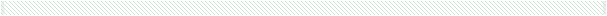CSC320W: Introduction to Visual ComputingSchedule and Notes
Lectures: W 6-8 pm (BA1190)
Tutorials W 8 pm
 Part I: Representing images as 2D arrays of pixels Week 1 Date Topic Details Readings Resources Wed, Jan 8 Introduction; The Camera Response Function Digital images; computing camera response functions from images. Slides: [Printer friendly] [Color] Sections 1.1-1.2, 2.1, 2.2, 2.4.2 (only paragraph entitled "silicon sensors"), 2.6.2 from Castleman book; Sections 1 and 2, up to Eq (2), from paper. As you read the paper, note that film response curve and camera response curve in the case of digital cameras, are one and the same. The HDRShop home page. Rendering with Natural Light (a movie that uses high-dynamic-range photography to capture outtdoor illumination and re-use it for image synthesis) No tutorial during week 1. Fri, Jan 10 Assignment Assignment 1 is out, due January 27. Week 2 Wed, Jan 15 Pixel components: color and alpha Color image acquisition; alpha matting and the matting equation    Slides: [pdf] Tutorial Matting and linear equations Part II: Representing images as continuous 1D and 2D functions Week 3 Wed, Jan 22 Computing 1D image derivatives Least-squares polynomial fitting; intensity derivatives; weighted least squares; RANSAC    Slides: [pdf] Polynomial fitting demo to be shown in next week's tutorial: polydemo.zip.  If you download it, run it for a variety of fits (LS, WLS, 1st degree, 2nd degree, etc) to see their effect. Tutorial Questions about Assignment 1 Week 4 Mon, Jan 27 Assignment Assignment 1 due at 11:59pm. Worth 10% of the final mark. Wed, Jan 29 Representing 2D image curves Local analysis of curves: the tangent & normal vectors, the moving frame    Slides: [pdf] To run the demo shown in class, unpack the zipfile curvedemo.zip You should run the demo for a variety of fits (LS, WLS, 1st degree, 2nd degree, etc) to see their effect on the estimated curve. Tutorial Polynomial fitting and 2D curves Tutorial notes on polynomial fitting and 2D curves by Micha Livne. Week 5 Wed, Feb 5 Edge detection Local analysis of 1D and 2D image patches: the Image Gradient. Case study: Painterly Rendering    Slides: [pdf] Paper by Litwinowicz on painterly rendering (this is not required reading). Tutorial Description of A2, Questions and answers about Assignment 1, part B [slides], and Paper on Accidental Pinhole and Pinspeck cameras (this is not required reading). Fri, Feb 7 Assignment Assignment 2 is out, due March 2 (two days later than originally advertised!). Week 6 Wed, Feb 12 Corner detection, Intelligent Scissors Relation between local shape near extrema and the eigenvectors/eigenvalues of the Hessian; relation between eigenvalues & the trace & determinant of a matrix; localizing edges as zero crossings of the Laplacian; the Lowe feature detector: finding non-cylindrical points through eigenvalue analysis of the Hessian of the Laplacian    Slides: [pdf] Paper by Mortensen on Intelligent Scissors (this is not required reading). This paper and the technique will be covered in one of the forthcoming tutorials. Tutorial Questions about Assignment 2 and a paper on Object Recognition by Pedro F. Felzenszwalb. [slides] Week 7 Wed, Feb 19 No lecture Reading week No tutorial Part III: Multi-resolution image representations Week 8 Wed, Feb 26 Template matching and correlation Representing images as vectors; evaluating similarity using RMS distance error, cross-correlation and normalized cross-correlation; Slides: [pdf] To run the demo shown in class, unpack the zipfile corrdemo.zip  and type corrdemo at the matlab prompt. Tutorial TBD Sun, Mar 02 Assignment Assignment 2 due at 11:59pm. Worth 10% of the final mark. Week 9 Wed, Mar 5 Principal Component Analysis Face recognition using Eigenfaces Slides: [pdf] Section 13.6 from Castleman To run the demo shown in class, unpack the zipfile recognition_demo.zip  and type pca_recdemo at the matlab prompt. Midterm BA 1190, 6 pm Worth 20% of the final mark Wed, Mar 5 Assignment Assignment 3 is out, due March 19. Part IV: Alternative image representations Week 10 Wed, Mar 12 Gaussian Pyramids The idea of representing an image at multiple resolutions is described and studied using Gaussian and Laplacian Pyramids. Slides: [pdf] Original paper by Burt and Adelson on the Gauss/Laplacian pyramids. You should read up to, but not including, section entitled Entropy. This Matlab demo shows how a 1D image changes as we smooth it with a sequence of Gaussians of increasing standard deviation Tutorial TBD Week 11 Wed, Mar 19 The Haar Wavelet Transform Wavelet compression of 1D and 2D images Slides: [pdf] A tutorial paper on the Haar Wavelets. Matlab wavelets demo shown in class. Wed, Mar 19 Assignment Assignment 3 due at 11:59pm. Worth 10% of the final mark. Wed, Mar 19 Assignment Assignment 4 is out, due April 2. Tutorial PCA, a few theoretical details. Week 12 Wed, Mar 26 Polynomial fitting vs. correlation; Matching images using SIFT Analysis of WLS polynomial fitting and image smoothing as a template matching operation; template matching expressed as a multiplication of an image with a Toeplitz matrix; Gaussian image smoothing; SIFT-based feature detection; the SIFT descriptor; image matching using SIFT Slides: [pdf] Sections 1-3 of paper by David Lowe describing SIFT. Web page on SIFT (with demo code) Tutorial On Morphing Slides: [pdf] Part V: Introduction to 2D Image Transformations Week 13 Wed, Apr 2 Homogeneous coordinates Homography-based image warping Slides: [pdf] Tutorial TBD Wed, Apr 4 Assignment Assignment 4 due at 11:59pm. Worth 10% of the final mark. Apr 9-30 Final Exam Location, time and date to be announced Worth 40% of the final mark